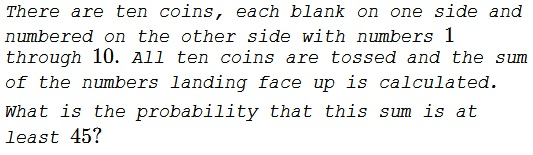# Random Sum

### Problem### Solution 1

The maximum possible sum is $1+2+\ldots+10=55.$ $45=55-10.$ It is easier to calculate the probability that the sum is at most than $10.$ This would be the sum of numbers written on the coins that fell face down. Just by inspection, there are $43$ combinations of numbers from $1$ to $10,$ each taken at most once, that sum to at most $10.$

### Solution 2

Sequence A000009 list, for a given index $N,$ the number of partitions of $N$ into distinct parts:

$\begin{array}{cccccccccccc} N&0&1&2&3&4&5&6&7&8&9&10\\ &1&1&1&2&2&3&4&5&6&8&10 \end{array}$

For example,

\begin{align} 7&=7+0=6+1=5+2=4+3\\ &=1+2+4\\ 8&=8+0=7+1=6+2=5+3\\ &=1+2+5=1+3+4. \end{align}

Summing up the eleven numbers in the second row of the above table gives $43,$ as above.

### Solution 3

The expansion of the generating function $\displaystyle \prod _{n\in S} \left(1+q^n\right)$ where $S$ is the set under consideration (here $1, 2, \dots, 10$), into polynomials, such as $a_1 q + a_2 q^2 \ldots +a_m q^m$ will produce coefficients that equal the number of distinct partitions of $m$.

We are considering values $\displaystyle 45 \leq j \leq\sum _{i=1}^{10} i=55:$

$\displaystyle\small{\prod _{i=1}^{10} \left(1+q^i\right)=q^{55}+q^{54}+q^{53}+2 q^{52}+2 q^{51}+3 q^{50}+4 q^{49}+5 q^{48}+6 q^{47}+8 q^{46}+10 q^{45}+\ldots},$

with coefficients $\{1,1,1,2,2,3,4,5,6,8,10\}$ that sum to $43$. The total number of tuples under consideration is $2^{10}$. Hence the probability is $\displaystyle \frac{43}{2^{10}}\approx .041992$.

### Solution 4### Acknowledgment

This is problem CC252 from the Canadian Crux Mathematicorum (V 44, NO. 1, January 2018). Originally Problem 4, Senior Round part B, of the 2014 BC Secondary Math Contest. Solution 1 has been posted by quite a few people. Solution 2 is by Mike Lawler; Solution 3 is by Robert Frey and N. N. Taleb; Solution 4 is by Humberto Barrios.Copyright © 1996-2018 Alexander Bogomolny

 64859750